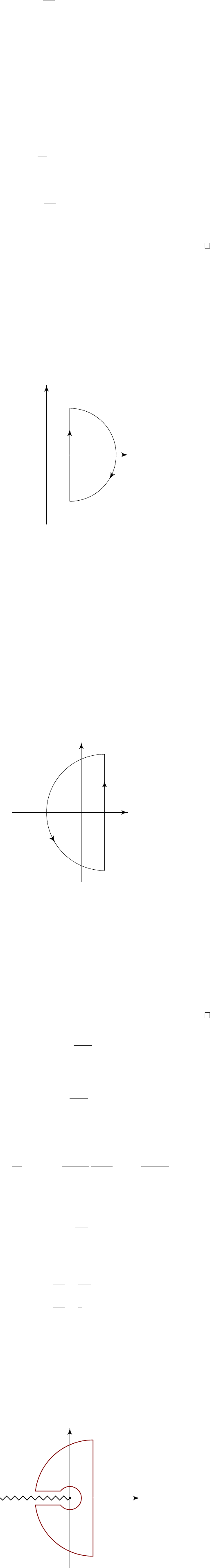5Transform theory

IB Complex Methods5.4 The inverse Laplace transform
It’s no good trying to find the Laplace transforms of functions if we can’t invert
them. Given
ˆ
f
(
p
), we can calculate
f
(
t
) using the Bromwich inversion formula.
Proposition. The inverse Laplace transform is given by
f(t) =
1
2πi
Z
c+i
ci
ˆ
f(p)e
pt
dp,
where
c
is a real constant such that the Bromwich inversion contour
γ
given by
Re p = c lies to the right of all the singularities of
ˆ
f(p).
Proof.
Since
f
has a Laplace transform, it grows no more than exponentially.
So we can find a c R such that
g(t) = f(t)e
ct
decays at infinity (and is zero for
t <
0, of course). So
g
has a Fourier transform,
and
˜g(ω) =
Z
−∞
f(t)e
ct
e
t
dt =
ˆ
f(c + ).
Then we use the Fourier inversion formula to obtain
g(t) =
1
2π
Z
−∞
ˆ
f(c + )e
t
dω.
So we make the substitution p = c + , and thus obtain
f(t)e
ct
=
1
2πi
Z
c+i
ci
ˆ
f(p)e
(pc)t
dp.
Multiplying both sides by
e
ct
, we get the result we were looking for (the re-
quirement that
c
lies to the right of all singularities is to fix the “constant of
integration” so that f (t) = 0 for all t < 0, as we will soon see).
In most cases, we don’t have to use the full inversion formula. Instead, we
use the following special case:
Proposition.
In the case that
ˆ
f
(
p
) has only a finite number of isolated singu-
larities p
k
for k = 1, ··· , n, and
ˆ
f(p) 0 as |p| , then
f(t) =
n
X
k=1
res
p=p
k
(
ˆ
f(p)e
pt
)
for t > 0, and vanishes for t < 0.
Proof.
We first do the case where
t <
0, consider the contour
γ
0
+
γ
0
R
as shown,
which encloses no singularities.
c iR
γ
0
c + iR
γ
0
R
×
×
×
Now if
ˆ
f(p) = o(|p|
1
) as |p| , then
Z
γ
0
R
ˆ
f(p)e
pt
dp
πRe
ct
sup
pγ
0
R
|
ˆ
f(p)| 0 as R .
Here we used the fact
|e
pt
| e
ct
, which arises from
Re
(
pt
)
ct
, noting that
t < 0.
If
ˆ
f
decays less rapidly at infinity, but still tends to zero there, the same
result holds, but we need to use a slight modification of Jordan’s lemma. So in
either case, the integral
Z
γ
0
R
ˆ
f(p)e
pt
dp 0 as R .
Thus, we know
R
γ
0
R
γ
. Hence, by Cauchy’s theorem, we know
f
(
t
) = 0 for
t <
0. This is in agreement with the requirement that functions with Laplace
transform vanish for t < 0.
Here we see why
γ
must lie to the right of all singularities. If not, then the
contour would encircle some singularities, and then the integral would no longer
be zero.
When t > 0, we close the contour to the left.
c iR
γ
0
c + iR
γ
R
×
×
×
This time, our
γ
does enclose some singularities. Since there are only finitely
many singularities, we enclose all singularities for sufficiently large
R
. Once
again, we get
R
γ
R
0 as R . Thus, by the residue theorem, we know
Z
γ
ˆ
f(p)e
pt
dp = lim
R→∞
Z
γ
0
ˆ
f(p)e
pt
dp = 2πi
n
X
k=1
res
p=p
k
(
ˆ
f(p)e
pt
).
So from the Bromwich inversion formula,
f(t) =
n
X
k=1
res
p=p
k
(
ˆ
f(p)e
pt
),
as required.
Example. We know
ˆ
f(p) =
1
p 1
has a pole at
p
= 1. So we must use
c >
1. We have
ˆ
f
(
p
)
0 as
|p|
. So
Jordan’s lemma applies as above. Hence
f
(
t
) = 0 for
t <
0, and for
t >
0, we
have
f(t) = res
p=1
e
pt
p 1
= e
t
.
This agrees with what we computed before.
Example.
Consider
ˆ
f
(
p
) =
p
n
. This has a pole of order
n
at
p
= 0. So we
pick c > 0. Then for t > 0, we have
f(t) = res
p=0
e
pt
p
n
= lim
p0
1
(n 1)!
d
n1
dp
n1
e
pt
=
t
n1
(n 1)!
.
This again agrees with what we computed before.
Example. In the case where
ˆ
f(p) =
e
p
p
,
then we cannot use the standard result about residues, since
ˆ
f
(
p
) does not vanish
as |p| . But we can use the original Bromwich inversion formula to get
f(t) =
1
2πi
Z
γ
e
p
p
e
pt
dp
=
1
2πi
Z
γ
1
p
e
pt
0
dp,
where
t
0
=
t
1. Now we can close to the right when
t
0
<
0, and to the left when
t
0
> 0, picking up the residue from the pole at p = 0. Then we get
f(t) =
(
0 t
0
< 0
1 t
0
> 0
=
(
0 t < 1
1 t > 1
= H(t 1).
This again agrees with what we’ve got before.
Example.
If
ˆ
f
(
p
) has a branch point (at
p
= 0, say), then we must use a
Bromwich keyhole contour as shown.